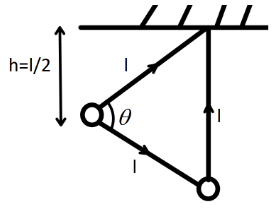Courses
Courses for Kids
Free study material
Free LIVE classes
More# A bob hangs from a rigid support by an inextensible string of length $l$. If it is displaced to a position whose displacement from the lowest position is $l$. Keeping the string straight and then released. The speed of the bob at the lowest position is: A. $\sqrt {gl}$ B. $\sqrt {3gl}$ C. $\sqrt {2gl}$ D. $\sqrt {5gl}$

Last updated date: 25th Mar 2023
Total views: 207.3k
Views today: 3.84kVerified
207.3k+ views
Hint:From conservation of energy find the speed of the bob at the lowest point. Conservation of energy states that the total mechanical energy of a system is conserved for a conservative force field.

Formula used:
The mechanical energy of a system in a conservative force field is constant,
$K.E + P.E = {\text{Constant}}$
where $K.E$ is the kinetic energy of the system and $P.E$ is the potential energy of the system.
Kinetic energy of a particle moving with velocity $v$ is,
$\dfrac{1}{2}m{v^2}$
where, $m$ is the mass of the particle.

We have given here the bob has a length of inextensible $l$ and it moves a displacement of $l$. So, now from moving to the initial position to a displacement of $l$ the potential energy and also the kinetic energy of the system will change. Since it moves from the lowest point to the highest point of its path the kinetic energy of the bob at the highest point will be zero and the potential energy at the lowest point will be zero.Since from vector sum we can see that,
${l^2} = {l^2} + {l^2} + 2{l^2}\cos \theta$
$\Rightarrow {l^2} = 2{l^2}(1 + \cos \theta )$
$\Rightarrow \dfrac{1}{2} = 1 + \cos \theta$
$\Rightarrow \cos \theta = - \dfrac{1}{2}$
Now, we can see that the change in height of the bob will be,
$h = l( - \dfrac{1}{2})$
taking the value of height only we have
$h = \dfrac{l}{2}$
Now, let kinetic energy at the lowest point be $\dfrac{1}{2}m{v^2}$.And the potential energy of the bob at the highest point is $mgh = mg\dfrac{l}{2}$. So, from conservation of energy we can write,
$mg\dfrac{l}{2} = \dfrac{1}{2}m{v^2}$
$\Rightarrow gl = {v^2}$
$\therefore v = \sqrt {gl}$
So, the velocity of the bob at the lowest point will be, $\sqrt {gl}$

Hence, option A is the correct answer.

Note:The gravitational force field is a conservational force field and since there is no dissipative force acting on the particle hence we can apply the conservation of energy or mechanical energy here. The negative sign of the height here implies that the height is decreased means the earlier point the bob was the lowest point.